# 玩转Zynq连载49——[ex68] MT9V034摄像头的图像FFT滤波处理

0# 2 基于Matlab的FFT滤波

使用project\zstar_ex68\matlab文件夹下的Matlab源码image_1D_fft_ifft.m或L1024_of_image_1D_fft_ifft.m（将640个点扩展为1024个点进行FFT变换，扩展的点以0填充，模拟FPGA的FFT IP核实际工作状况），对测试图像test进行FFT变换，进行必要的滤波，然后IFFT逆变换。

测试图像为彩色图像，原始图像如下。首先进行彩色转灰度的变换，灰度图像如下。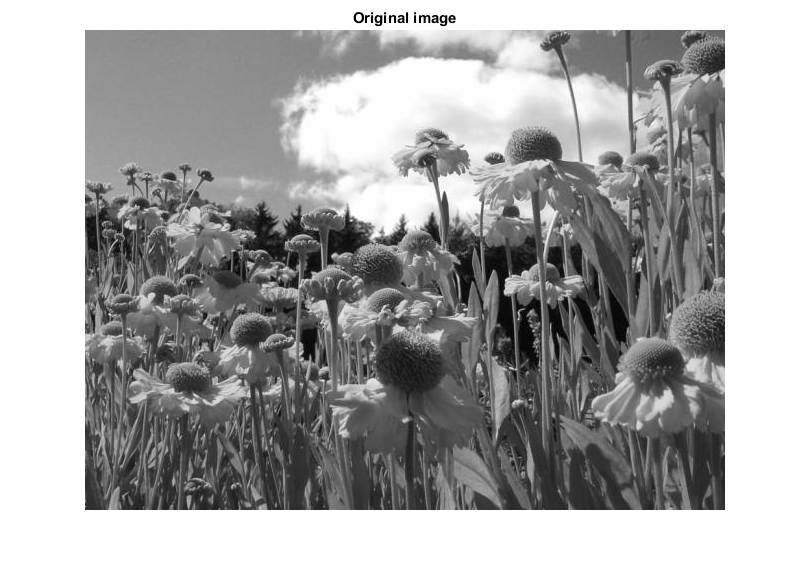提取出其中1行进行FFT变换后的图像频谱如下。很明显，大部分高频分量集中在前面几个点，而后面的点几乎频率都很小。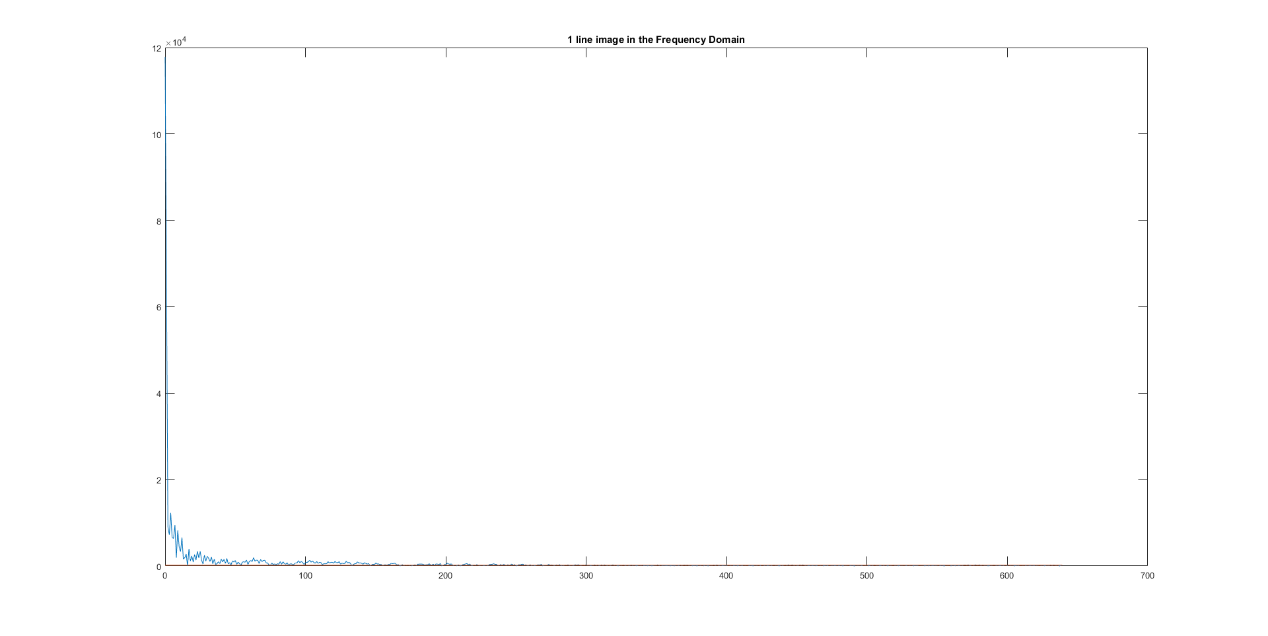放大频谱图，看到细节如下。这里绘制了一条取值为300的直线，有将近50%的频谱集中在这条线以下。若是做图像压缩，其实我们可以把这些低频分量忽略了，那么数据量可能会大大降低，当然了，副作用是图像可能会有一定程度的失真，有失必有得嘛。滤除这些低频分量，也会使图像更锐一些。话说做FFT变换的目的可远不止这些，在一些特殊的应用场景中，我们总是希望从原始图像中提取出一些和应用直接相关的特征信息，那么做了FFT后的图像常常非常有益于这些操作。为了演示，这里我们的代码里面就将这些低于300的点都滤除，即取0。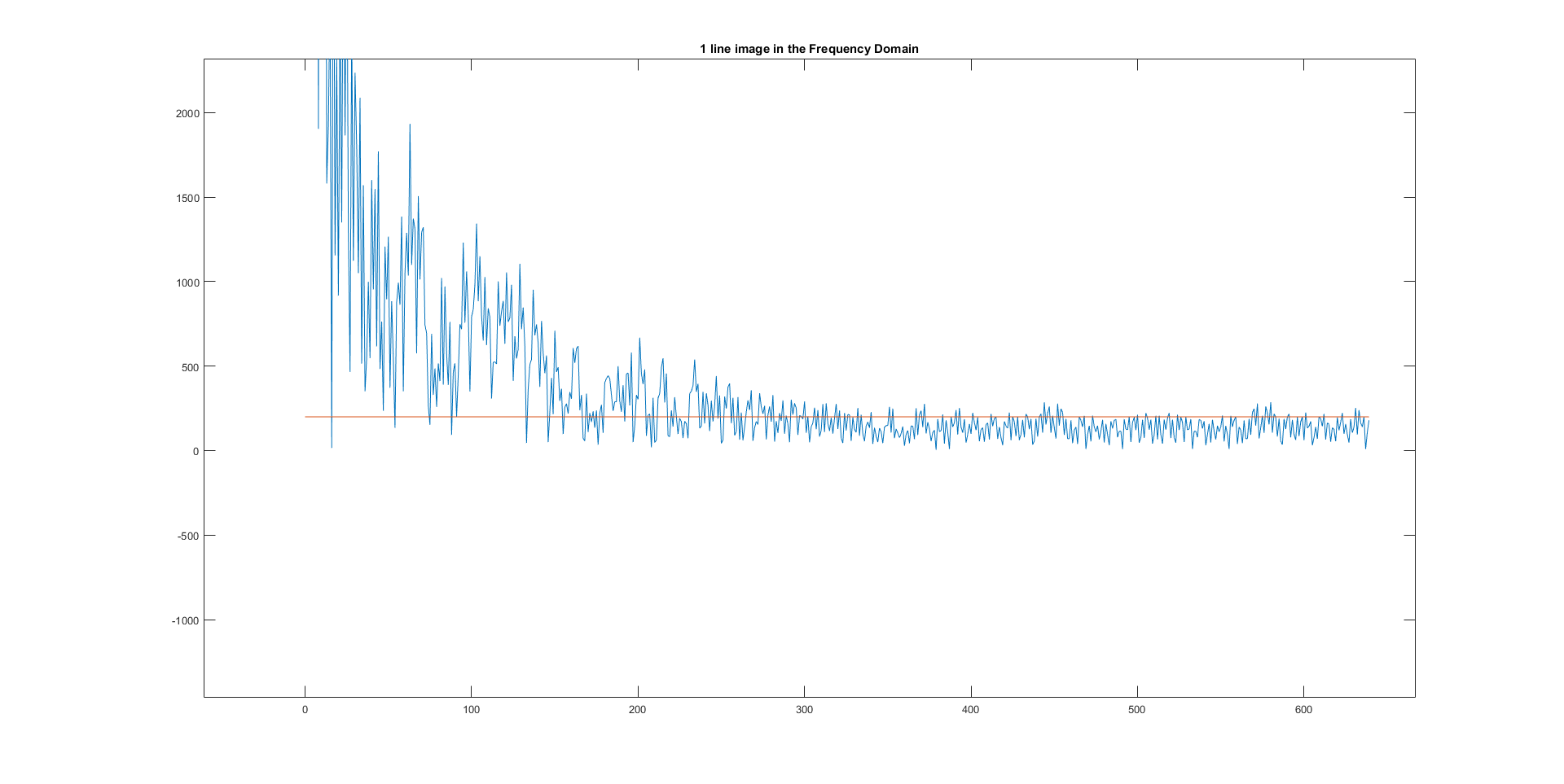从频谱图上看，如图所示，右侧的滤波后明显图像偏黑（很多值取0了）了。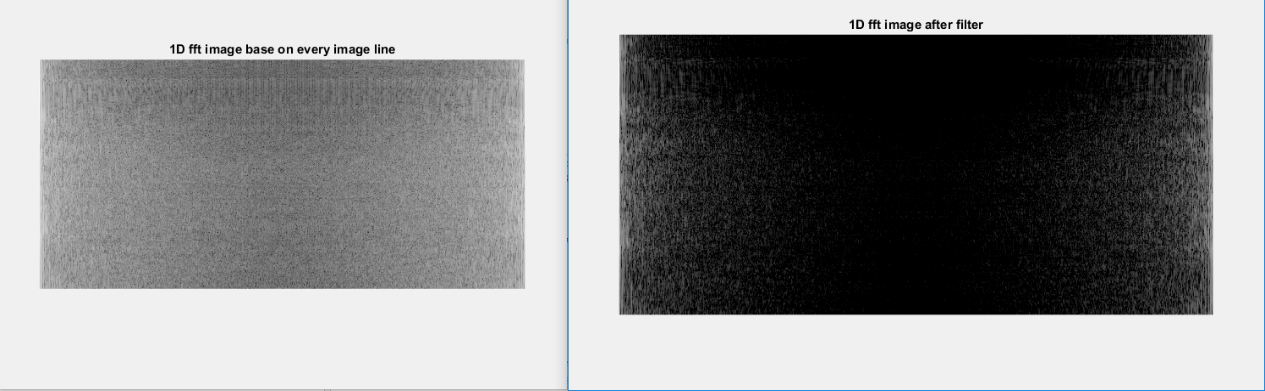我们重新把原图放到这里，和FFT滤波并IFFT以后的图像做比对，图像整体仍然保持不变，但是查看细节，可以发现处理后的图像明显锐了一些。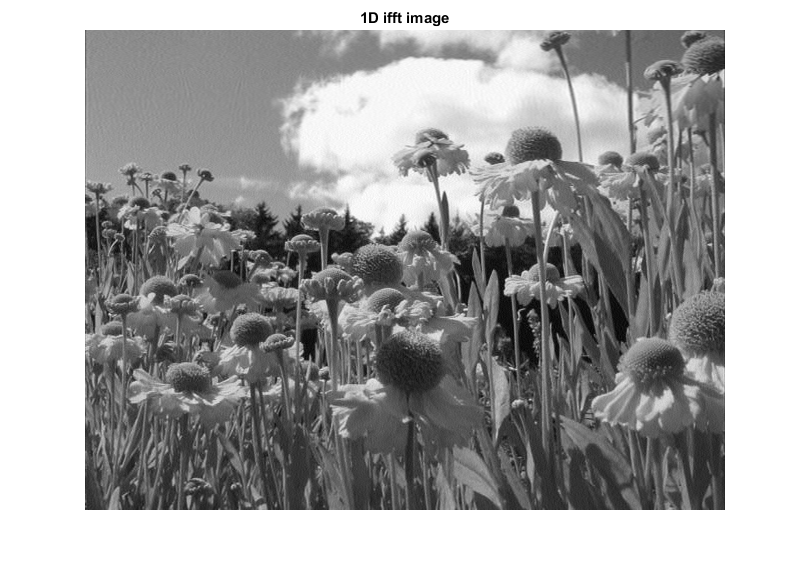Matlab源码如下：

clc;clear `all;close all;

IMAGE_WIDTH = 640;

IMAGE_HIGHT = 480;

I = rgb2gray(I);

%fclose(fid1);

%% output image data in hex file

raw_image = reshape(I, IMAGE_HIGHT, IMAGE_WIDTH);

raw_image = raw_image';

fid2 = fopen('image_in_hex.txt', 'wt');

fprintf(fid2, '%04x\n', raw_image);

fid2 = fclose(fid2);

%show origin image

figure,imshow(I);

title('Original image');

%1D fft base on every image line

II = zeros(IMAGE_HIGHT,1024);

J = zeros(IMAGE_HIGHT,1024);

for i = 1:IMAGE_HIGHT

for j = 1:IMAGE_WIDTH

II(i,j) = I(i,j);

end

J(i,:) = fft(II(i,:));%fft(I(i,:));

end

%show 1 line fft result

t1 = (0:IMAGE_WIDTH);                % Time vector

line = ones(IMAGE_WIDTH) * 200;

figure;

plot(t1(1:IMAGE_WIDTH),abs(J(50,1:IMAGE_WIDTH)),t1(1:IMAGE_WIDTH),line(1:IMAGE_WIDTH))

title(['1 line image in the Frequency Domain'])

%show fft of origin image

figure,imshow(log(abs(J)),[]);

title('1D fft image base on every image line');

%colormap(jet(64)),colorbar;

%fft fiter

J(abs(J) < 300) = 0;

%J(abs(J) > 1000) = 1000;

%show fft of fft filter image

figure,imshow(log(abs(J)),[]);

title('1D fft image after filter');

%1D ifft base on every image line

K = zeros(IMAGE_HIGHT,1024);

for i = 1:IMAGE_HIGHT

K(i,:) = real(ifft(J(i,:)));

end

KK = zeros(IMAGE_HIGHT,IMAGE_WIDTH);

for i = 1:IMAGE_HIGHT

for j = 1:IMAGE_WIDTH

KK(i,j) = K(i,j);

end

end

%show ifft image

figure,imshow(KK,[])

title('1D ifft image');

# 3 FPGA仿真

在Sources面板中，展开Simulation Sources à sim_1，将sim_fft.v文件设置为top module。同样是对前面的测试图像,经过FFT和IFFT变换后存储在image_view0.txt文本中（仿真测试结果位于project\zstar_ex68\zstar.sim\sim_1\behav文件夹下）。为了确认FFT和IFFT IP核运算的精度和效果，这里没有做任何的滤波处理。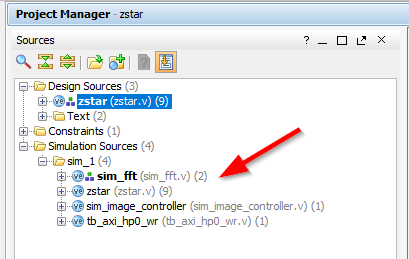使用draw_image_from_FPGA_result.m脚本（project\zstar_ex68\ matlab文件夹下）导入image_view0.txt文本的图像，和原始图像比对如下所示。看到图像几乎没有任何失真。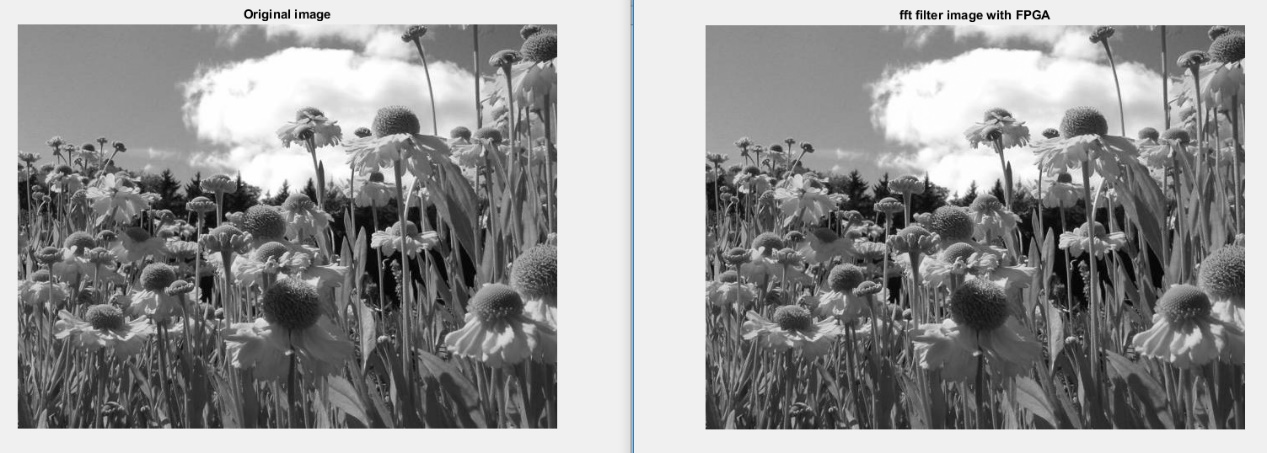# 4 基于FPGA的图像平滑处理

工程文件夹project\zstar_ex688\zstar.srcs\sources_1\new下的image_fft_filter.v模块以及3个子模块image_fft_controller.v、image_filter.v和image_ifft_controller.v实现了图像的FFT变换、滤波和IFFT变换处理。FPGA设计的功能框图如下。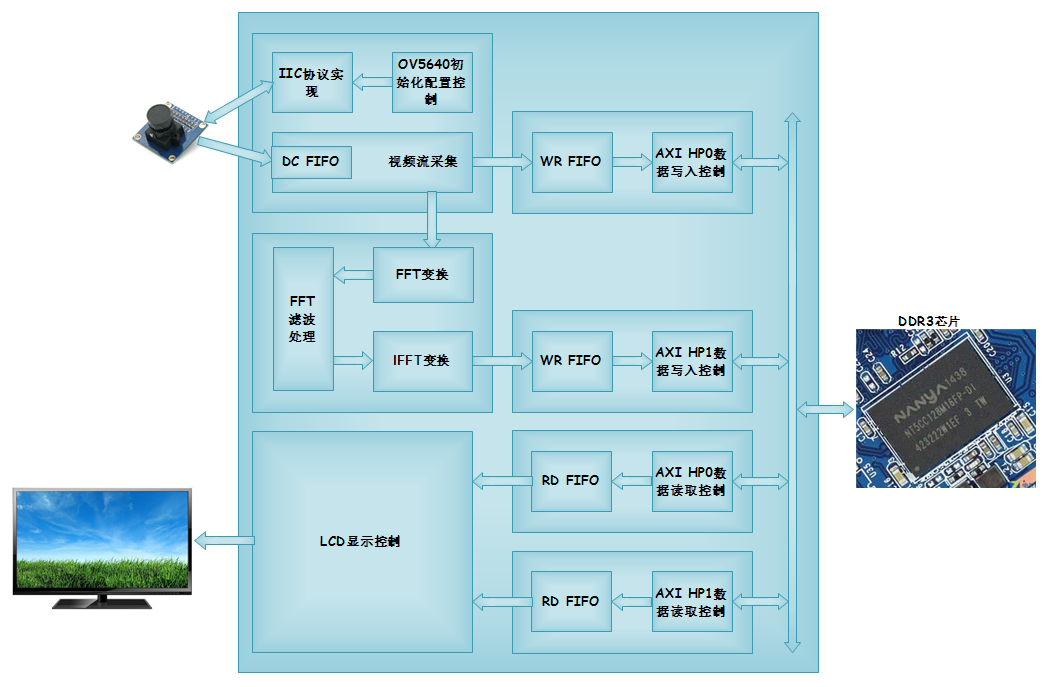image_fft_controller.v模块例化FFT IP核，将采集的图像留以行为单位输入到FFT IP核，输出FFT频域数据。

image_filter.v模块对FFT频域数据计算绝对值并进行必要的滤波处理，假设FFT结果的实部值为a，虚部值为b，那么其绝对值abs =sqrt(a^2+b^2)。如下代码，注释部分可以滤除低频分量，当前例程中为了验证FFT和IFFT变换后精度没有损失，未作滤波。

always @(posedge clk or negedge rst_n)

if(!rst_n) begin

o_image_filter_data_image <= 20'd0;

o_image_filter_data_real <= 20'd0;

end

/*else if(sqrt_fft[19:0] < 20'd300) begin //此处可以做必要的高频或低频滤波处理

o_image_filter_data_image <= 20'd0;

o_image_filter_data_real <= 20'd0;

end*/

else begin

o_image_filter_data_image <= r_image_fft_data_image[TOTAL_LATENCY-1];

o_image_filter_data_real <= r_image_fft_data_real[TOTAL_LATENCY-1];

end

image_ifft_controller.v模块将滤波处理后的FFT结果进行IFFT变换，图像转回时域值，供后续模块缓存DDR3并显示。

# 5 装配说明

MT9V034摄像头模块通过Zstar ISB底板（P3）与Zstar Zynq开发板连接，VGA也是通过Zstar ISB底板与Zstar Zynq开发板连接，VGA板同时需要连接到VGA显示器。连接示意如图所示。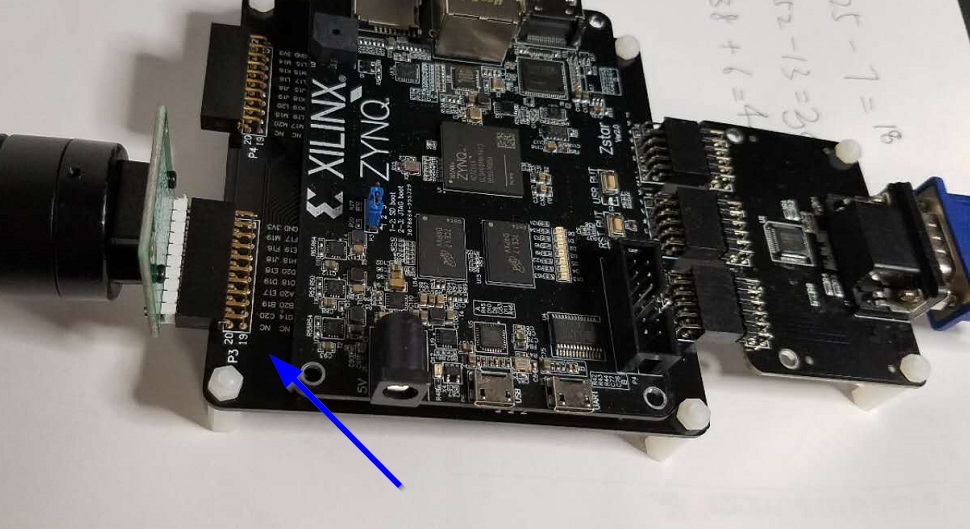# 6 板级调试

本实例对应ex68实例工程，已经制作好的BOOT.bin放置在工程路径“zstar_ex68\zstar.sdk\BOOT”下。也可以参考文档《玩转Zynq-实例篇：[ex51] 制作裸跑程序的启动文件BOOT.bin.pdf》制作包含.bit文件的BOOT.bin文件，将其拷贝到TF卡中，插入Zstar开发板的卡槽中，做好装配连接，上电，可以看到VGA显示器同时显示左右两个图像，左侧图像为原始图像，右侧图像为经过FFT和IFFT后还原的图像。